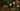# JavaScript ES6 解构举例教程

JavaScript 解构是一种赋值语法表达，简洁且可读地“解构”数组的值或者对象的属性，解析为不同的变量。

## JavaScript 解构表达式(例子)

``````[a, b] = [50, 100];

console.log(a);
// expected output: 50

console.log(b);
// expected output: 100

[a, b, ...rest] = [10, 20, 30, 40, 50];

console.log(rest);
// expected output: [30, 40, 50]``````

TLDR;

## 数组解构

### 基础变量赋值

``````let foo = ['one', 'two', 'three'];

let [red, yellow, green] = foo;
console.log(red); // "one"
console.log(yellow); // "two"
console.log(green); // "three"``````

### 声明分别赋值

``````// 声明变量
let a, b;
// 然后分别赋值
[a, b] = [1, 2];
console.log(a); // 1
console.log(b); // 2``````

### 解构默认值

``````let a, b;
// 设置默认值
[a = 5, b = 7] = ;
console.log(a); // 1
console.log(b); // 7``````

### 交换变量值

``````let a = 1;
let b = 3;

[a, b] = [b, a];
console.log(a); // 3
console.log(b); // 1``````### 解析函数返回的数组

``````function c() {
return [10, 20];
}

let a, b;
[a, b] = c();
console.log(a); // 10
console.log(b); // 20``````

### 忽略返回值/跳过某项

``````function c() {
return [1, 2, 3];
}

let [a, , b] = c();
console.log(a); // 1
console.log(b); // 3``````

``[, ,] = c();``

### 赋值数组剩余值给一个变量

``````let [a, ...b] = [1, 2, 3];
console.log(a); // 1
console.log(b); // [2, 3]``````

``````let [a, ...b,] = [1, 2, 3];
// SyntaxError: rest element may not have a trailing comma``````

### 嵌套数组解构

``````const color = ['#FF00FF', [255, 0, 255], 'rgb(255, 0, 255)'];

// Use nested destructuring to assign red, green and blue
// 使用嵌套解构赋值 red, green, blue
const [hex, [red, green, blue]] = color;

console.log(hex, red, green, blue); // #FF00FF 255 0 255``````

## 对象解构

### 基础对象解构

``````let x = { y: 22, z: true };
let { y, z } = x;

console.log(y); // 22
console.log(z); // true``````

### 无声明解构

``````let y, z;

({ y, z } = { y: 1, z: 2 });``````

`{a, b} = {a: 1, b: 2}` 不是有效的独立语法，左侧的 `{a, b}` 被考虑为代码块而不是一个对象字面量。

`(...)` 表达式需要前置分号或者它可能用于在前一行执行函数。

### 赋值给新变量名

``````let o = { p: 22, q: true };
let { p: foo, q: bar } = o;

console.log(foo); // 22
console.log(bar); // true``````

### 解构默认值

``````let { a = 10, b = 5 } = { a: 3 };

console.log(a); // 3
console.log(b); // 5``````

### 赋值给新变量名的同时提供默认值

``````let { a: aa = 10, b: bb = 5 } = { a: 3 };

console.log(aa); // 3
console.log(bb); // 5``````

### 嵌套对象和数组解构

``````const metadata = {
translations: [
{
locale: 'de',
localization_tags: [],
last_edit: '2014-04-14T08:43:37',
title: 'JavaScript-Umgebung',
},
],
};

let {
title: englishTitle, // 重命名
translations: [
{
title: localeTitle, // 重命名
},
],

console.log(localeTitle); // "JavaScript-Umgebung"``````

### 可计算对象属性名与解构

``````let key = 'z';
let { [key]: foo } = { z: 'bar' };

console.log(foo); // "bar"``````

### 同时使用数组和对象解构

``````const props = [
{ id: 1, name: 'Fizz' },
{ id: 2, name: 'Buzz' },
{ id: 3, name: 'FizzBuzz' },
];

const [, , { name }] = props;

console.log(name); // "FizzBuzz"``````

``````let x = [1, 2, 3, 4, 5];
let [y, z] = x;
console.log(y); // 1
console.log(z); // 2``````